## Series Solutions: Hermite's Equation#### Exercise 2:

Find the Hermite Polynomials of order 2, 4 and 6.

Recall that the recurrence relations are given by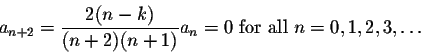We have to evaluate these coefficients for k=2, k=4 and k=6, with initial conditions a0=1, a1=0.

When k=2,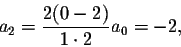while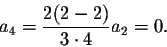Consequently all even coefficients other than a2 will be zero. Since a1=0, all odd coefficients will be zero, too. Thus

H2(t)=1-2t2.

When k=4,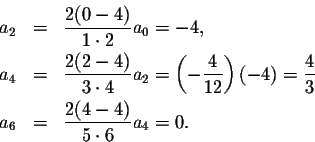Consequently all even coefficients other than a2 and a4 will be zero. Since a1=0, all odd coefficients will be zero, too. Thus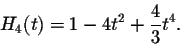You can check that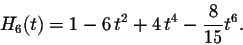[Back] [Exercises] [Next]
[Algebra] [Trigonometry] [Complex Variables]
[Calculus] [Differential Equations] [Matrix Algebra]S.O.S MATHematics home page

Do you need more help? Please post your question on our S.O.S. Mathematics CyberBoard.Helmut Knaust
1998-07-08